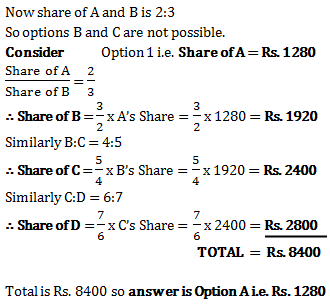# Ratio and Proportion - Quantitative Aptitude (MCQ) questions for Q. 29300

Q.  Rs. 8400 is divided among A, B, C and D in such a way that the shares of A and B, B and C, and C and D are in the ratios of 2:3, 4:5 and 6:7 respectively. The share of A is
- Published on 11 Apr 17

a. Rs. 1280
b. Rs. 8400
c. Rs. 8210
d. Rs. 1320#### Discussion

• Abhishek Sharma   -Posted on 19 May 18
That's a very lame and unprofessional method to solve this question.
A:B =2:3 and B:C =4:5
Therefore A:B:C =8:12:15
Also C:D=6:7
Therefore A:B:C:D=16:24:30:35
SHARE OF A= 16/105 ×8400
=? 1280

## ➨ Post your comment / Share knowledgeEnter the code shown above:

(Note: If you cannot read the numbers in the above image, reload the page to generate a new one.)